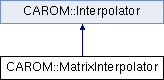libROM  v1.0 Data-driven physical simulation library
CAROM::MatrixInterpolator Class Reference

`#include <MatrixInterpolator.h>`

Inheritance diagram for CAROM::MatrixInterpolator:## Public Member Functions

MatrixInterpolator (std::vector< Vector * > parameter_points, std::vector< Matrix * > rotation_matrices, std::vector< Matrix * > reduced_matrices, int ref_point, std::string matrix_type, std::string rbf="G", double epsilon=1.0)
Constructor. More...

Matrixinterpolate (Vector *point)
Obtain the interpolated reduced matrix of the unsampled parameter point. More...

## Additional Inherited MembersProtected Member Functions inherited from CAROM::Interpolator
Interpolator (std::vector< Vector * > parameter_points, std::vector< Matrix * > rotation_matrices, int ref_point, std::string rbf, double epsilon=1.0)
Constructor. More...

std::vector< double > obtainRBFToTrainingPoints (Vector *point)
Compute the RBF from the parameter points with the unsampled parameter point. More...

double obtainRBF (Vector *point1, Vector *point2)
Compute the RBF between two points. More...Protected Attributes inherited from CAROM::Interpolator
int d_rank
The rank of the process this object belongs to.

int d_num_procs
The number of processors being run on.

int d_ref_point
The index within the vector of parameter points to the reference point.

std::string d_rbf

int d_epsilon
The RBF parameter that determines the width of influence. a small epsilon: larger influential width a large epsilon: smaller influential width.

std::vector< Vector * > d_parameter_points
The sampled parameter points.

std::vector< Matrix * > d_rotation_matrices
The reduced bases with compatible coordinates.

Matrixd_lambda_T
The reduced elements in tangential space.

## Detailed Description

MatrixInterpolator interpolates reduced matrices of a set of parameter points and returns an interpolated reduced matrix for an unseen parameter point. The performance of this algorithm depends on the matrix sizes being small because of its computational complexity due to direct matrix inversion. The interpolation algorithm was adapted from "Gradient-based Constrained Optimization Using a Database of Linear Reduced-Order Models" by Y. Choi et al.

## Constructor & Destructor Documentation

 CAROM::MatrixInterpolator::MatrixInterpolator ( std::vector< Vector * > parameter_points, std::vector< Matrix * > rotation_matrices, std::vector< Matrix * > reduced_matrices, int ref_point, std::string matrix_type, std::string rbf = `"G"`, double epsilon = `1.0` )

Constructor.

Parameters
 [in] parameter_points The parameter points. [in] rotation_matrices The rotation matrices associated with each parameter point. [in] reduced_matrices The reduced matrices associated with each parameter point. [in] ref_point The index within the vector of parameter points to the reference point [in] matrix_type The type of matrix (R = real, B = basis [also a real matrix, but uses a unique rotation specific to bases (AQ)], NS = nonsingular, SPD = symmetric positive-definite) [in] rbf The RBF type ("G" == gaussian, "MQ" == multiquadric, "IQ" == inverse quadratic, "IMQ" == inverse multiquadric) [in] epsilon The RBF parameter that determines the width of influence.

## Member Function Documentation

 Matrix* CAROM::MatrixInterpolator::interpolate ( Vector * point )

Obtain the interpolated reduced matrix of the unsampled parameter point.

Parameters
 [in] point The unsampled parameter point.

The documentation for this class was generated from the following file: